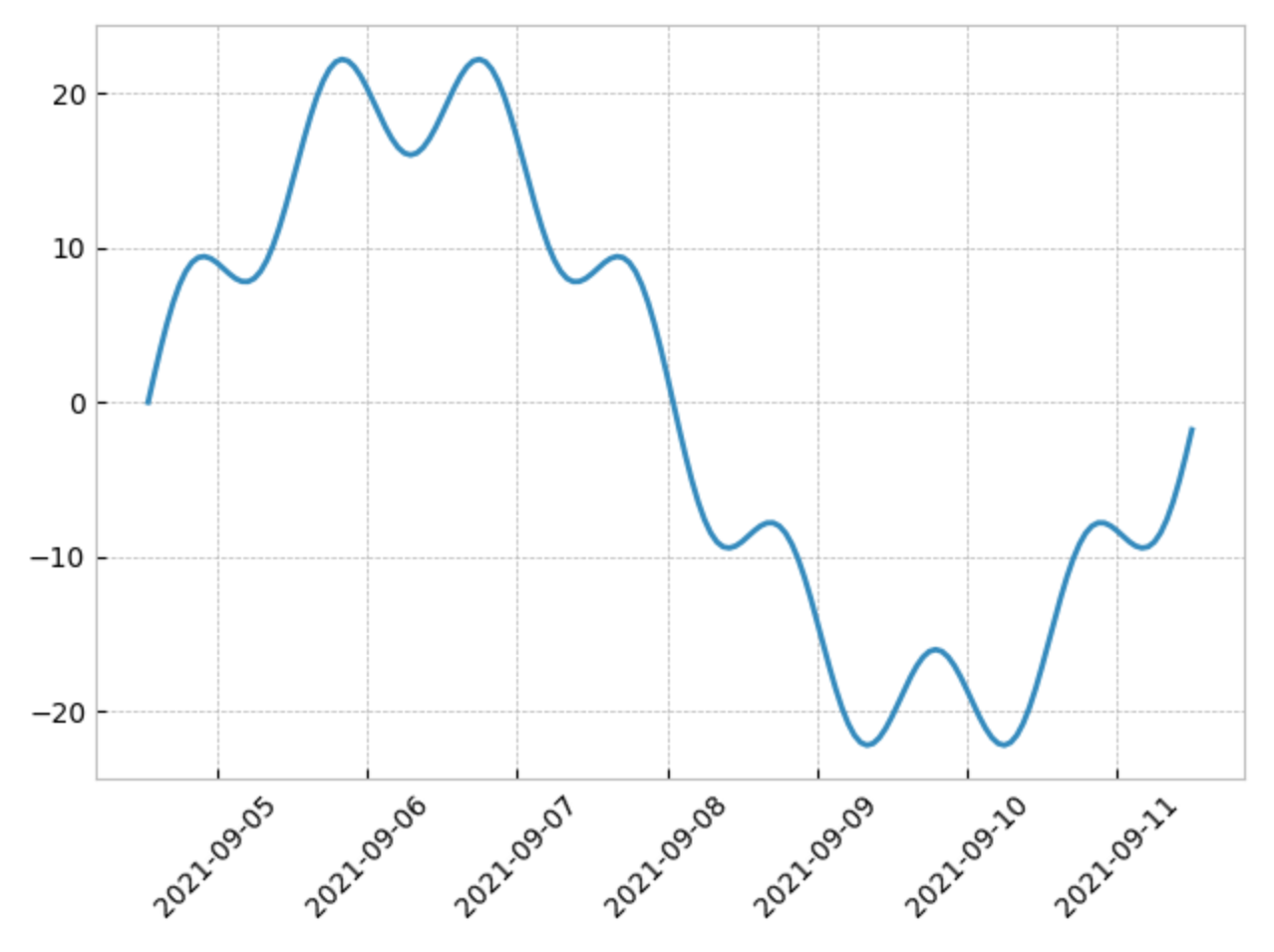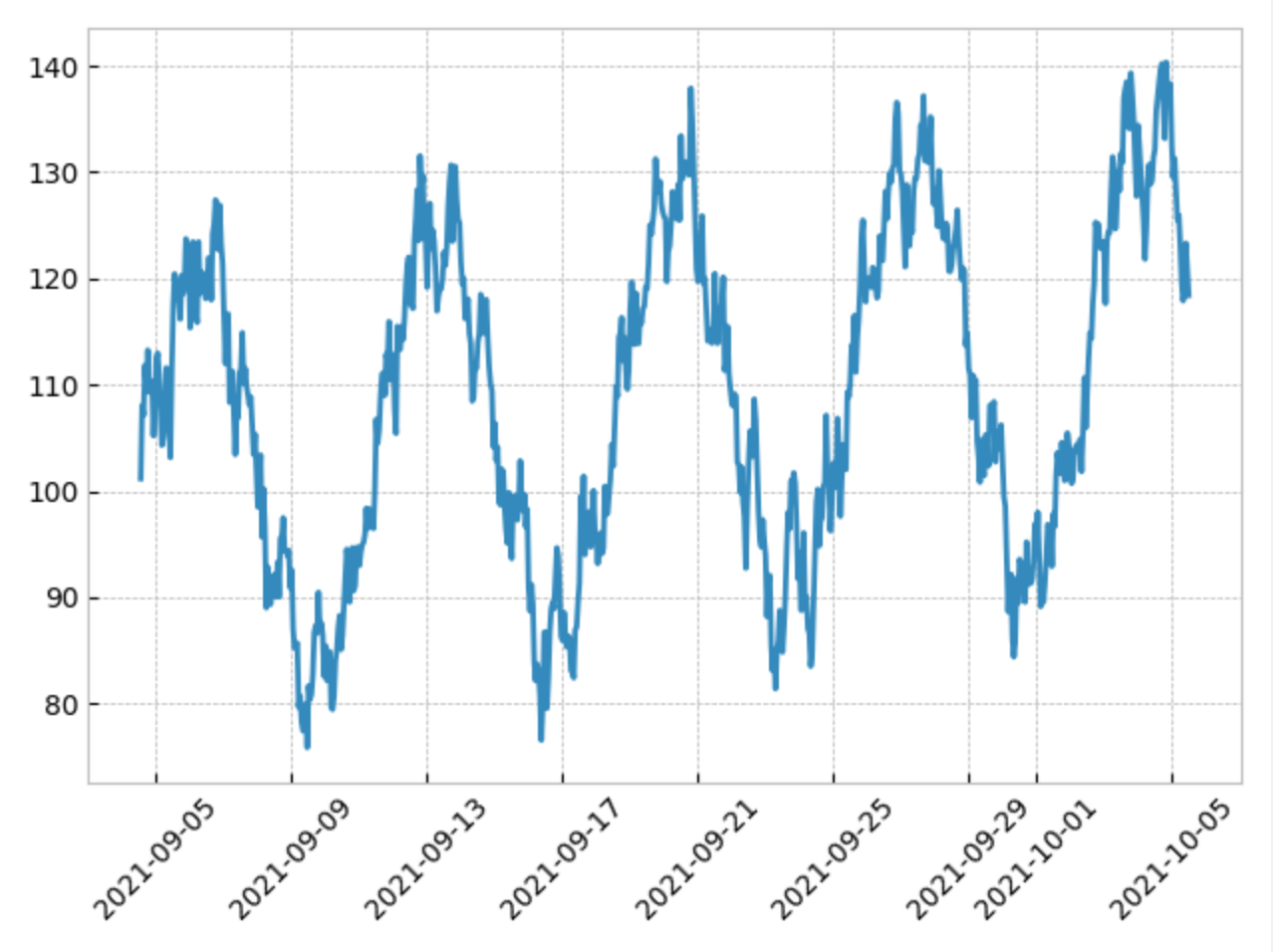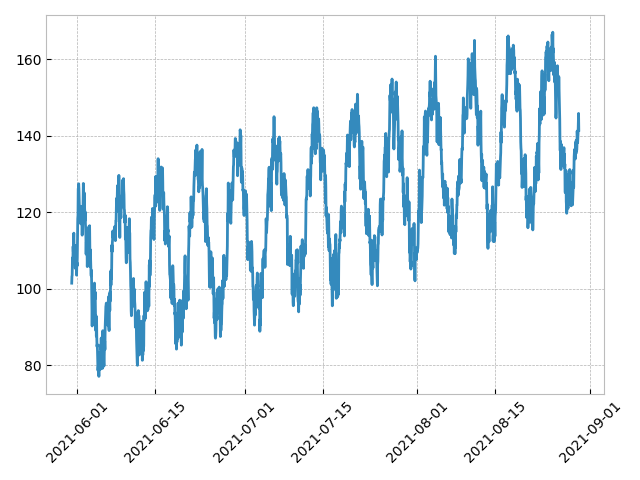# Getting Started

Let's discover mockseries in less than 5 minutes 🚀

## 1. Install mockseries#

pip install mockseries

## 2. Create a timeseries generator#

A timeseries can be expressed as a combination of 3 components: trend, seasonality and noise.

The trend is the long term, average change of the timeseries, such as the increase in CO2 emissions.
Seasonality is a cyclic pattern in the timeseries, such as the impact of the day-night cycle.
Noise represents irregular and random changes of the timeseries.

from datetime import timedelta
from mockseries.trend import LinearTrend
from mockseries.seasonality import SinusoidalSeasonality
from mockseries.noise import RedNoise
trend = LinearTrend(coefficient=2, time_unit=timedelta(days=4), flat_base=100)
seasonality = SinusoidalSeasonality(amplitude=20, period=timedelta(days=7)) \
+ SinusoidalSeasonality(amplitude=4, period=timedelta(days=1))
noise = RedNoise(mean=0, std=3, correlation=0.5)
timeseries = trend + seasonality + noise

Here, 2 seasonalities with a different period are combined. It's as easy as an addition !
Many types of trends, seasonalities and noises are available.
Just combine them with the operators +, - and *.

## 3. Preview#

# preview on minute, hour, day, month, year
seasonality.preview_week()
timeseries.preview_month()
##<style="width:100%; aspect-ratio:9/6;" src="">## 4. Generate values#

Sample your timeseries on a timeframe.

from datetime import datetime
from mockseries.utils import datetime_range
time_points = datetime_range(
granularity=timedelta(hours=1),
start_time=datetime(2021, 5, 31),
end_time=datetime(2021, 8, 30),
)
ts_values = timeseries.generate(time_points=time_points)

`datetime_range` helps you get the time points of a given granularity in a timeframe.
For instance:
timeframe of 1 hour, with a granularity of 1 minute: 60 points;
timeframe of 1 hour, with a granularity of 1 second: 3600 points.

## 5. Plot or write to csv#

from mockseries.utils import plot_timeseries, write_csv
plot_timeseries(time_points, ts_values)
write_csv(time_points, ts_values, "hello_mockseries.csv")

You will get something like this: